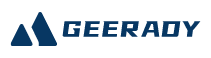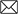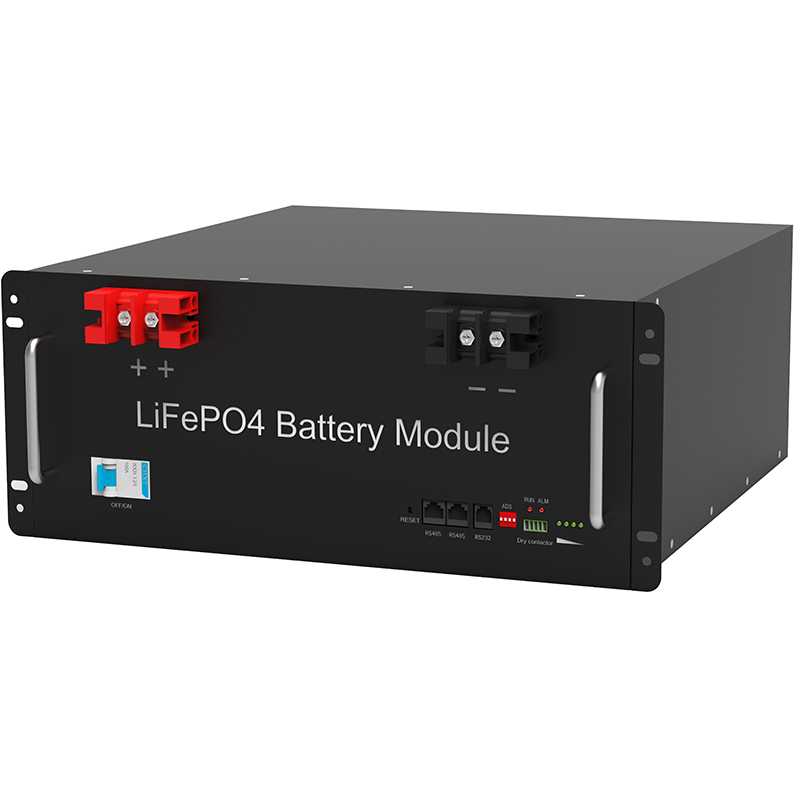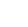#Emial:sales@geerady.com
Location： > > Comprehensive application plan for photovoltaic solar storage battery

### Comprehensive application plan for photovoltaic solar storage battery

Return Listsource：Gratene date： 2022-06-17Through the unremitting efforts of photovoltaic workers, the production technology of solar cells has been continuously improved and is increasingly used in various fields. In particular, in terms of post and telecommunications, due to the rapid development of the communication industry in recent years, the requirements for communication power supply are also increasing, so stable and reliable solar power supplies are widely used in communication. How to design the economical and reliable photovoltaic power system in accordance with the solar radiation conditions in various regions, which is a long-awaited topic for many experts scholars, and many excellent research results have laid the development of my country’s photovoltaic work. solid foundation.

When studying each expert’s design method, these design only considered the self-sustained time of the battery (that is, the longest continuous rainy day), but did not take into account the shortest recovery time of the battery after the loss (ie, the longest continuous rain in the two sets). The minimum number of days between days). This problem should be highly valued, especially in the southern part of my country, because there are many rainy days in the southern part of my country, and for the convenience of separate photovoltaic power systems, this issue should be included in the design because there is no emergency use of other power supply protection. Consider together.

1. This paper integrates the advantages of the previous design methods, combining the author’s experience in the design of photovoltaic power system for many years, introducing two groups of the shortest interval between the two sets of continuous rainy days as one of the requirements of design, and considering Various factors affecting solar radiation conditions, propose the calculation formula of solar cells, battery capacity, and related design methods.
2. Many factors affecting design
The solar light of the radiation light on the ground solar cell, the light intensity is affected by the thickness of the atmosphere (ie atmospheric mass), geographic position, the climate and meteorological, terrain object, etc., and its energy is on one day, January And there is a big change in the year, and even the total amount of radiation every year has a big difference.
The photoelectric conversion efficiency of the solar cell formar is affected by the temperature, sunlight and battery voltage floating, and the three of the three can change, so the photoelectric conversion efficiency of the solar cell format is also variables.
The battery pack is also working in a floating charge state, and its voltage varies with the change in power generation and load electricity. The energy provided by the battery is also affected by the ambient temperature.
Solar battery charge and discharge controller is manufactured by electronic components, it also needs energy consumption, while the performance, quality, etc. of the components used are also related to the size of the energy consumption, which affects the efficiency of charging.
The electricity of the load is also depending on the use, such as communication relay station, unmanned weather station, etc., has a fixed device power consumption. Some equipment such as lighthouse, navigation light, civil lighting and live electricity and other equipment, and electricity are often changed.
Therefore, the design of the solar power supply system needs to be considering more complicated factors. Features: The data used is mostly data, and the measurement of each statistics, and the choice of data is important.
The designer’s task is: under the environmental conditions of the solar cells (ie, the location of the location, solar radiation energy, climate, weather, terrain, etc.), the design of solar cells and battery power systems To pay attention to economic benefits, it is necessary to ensure the high reliability of the system.
The solar radiant energy data of a particular location is based on the information provided by the meteorological station for designing the solar cell. These meteorological data need to accumulate the average of even decades.
The period on the earth is irradiated with sunlight and the cycle of radiation can be a day 24h. The power generation of the solar cells in a certain area also has a periodic change of 24h, which is the same as the variation of the radiation in the region. However, the changes in the weather will affect the amount of power generation. If there is a few days of continuous rainy days, the square causing almost unable to power generation, can only be powered by the battery, while the battery depth is refined to add it as soon as possible. Most of the designers have the main data of the design of the design of the sun daily radiation energy or annual sunshine time provided by the sun. Due to the different years of data in a region, the minimum data should be taken in the near 10 years. Depending on the power consumption of the load, the battery is required to be powered in the sunshine and no sun. Solar radiation or total sunshine hours provided by meteorological stations are indispensable to determine the capacity size of the battery.
For solar cells, the load should include all power consumption devices in the system (except for the electrical storage and battery and lines, controllers, etc.).
The output power of the array is related to the number of components string, and the series is in order to obtain the desired operating voltage, in order to obtain the desired operating current, the appropriate number of components have been subjected to the required solar cell.
3 battery pack capacity design
The energy storage device of the solar cell power system is mainly a battery. Battery with solar cell formation is usually operated in a floating state, and its voltage varies with changes in power generation and load electricity. Its capacity is much larger than the power required for the load. The energy provided by the battery is also affected by the ambient temperature. In order to match solar cells, it is required to have a long service life and simple maintenance.
(1) Selection of batteries
There are many types of batteries that can be used with solar cells. It is currently widely used in lead-acid-free maintenance storage batteries, ordinary lead-acid batteries and alkaline nickel-cadmium storage batteries. At present, lead-acid-free maintenance battery is currently used because of its inherent “exemption” maintenance characteristics and the characteristics of less environmental contamination, it is suitable for useful solar power systems, especially unattended workstations. Ordinary lead-acid batteries are mainly suitable for maintenance capacity or low-end applications due to frequent maintenance and their environmental pollution. Although alkaline nickel-cadmium batteries have better low temperature, overtake, overproduction, but because of their high prices, it is only suitable for more special occasions.
(2) Calculation of battery pack capacity
The capacity of the battery is important for ensuring continuous power. In one year, the square array power generation has a big difference. The power generation of the square array does not satisfy the month desired, and the electric energy of the battery is to make up; in the month exceeding the month, the excess electrical energy is stored by the battery. Therefore, the shortcomings of the square array power generation and excessive value are one of the basis for determining the capacity of the battery. Similarly, the load electrical electricity during continuous rainy days must also be obtained from the battery. Therefore, the power consumption during this period is also one of the factors that determine the capacity of the battery.
Therefore, the capacity BC calculation formula of the battery is:
BC = a × ql × nl × to / ccah (1)
Where: A is a safety factor, take 1.1 to 1.4;
QL is average power consumption on load daily, and multiplies the working current by day;
NL is the maximum number of continuous rain days;
TO is a temperature correction coefficient, typically at 0 ° C, 1, and above -10 ° C is removed from 1.2 or less.
The CC is a depth of battery discharge, and generally lead-acid batteries take 0.75, alkaline nickel-cadmium battery takes 0.85.
4. Solar battery square array design
(1) Solar cell module series NS
The solar cell module can be connected in series, and the required operating voltage can be obtained, but the series of series of solar cell modules must be appropriate. The number of series is too small, and the series voltage is lower than the battery floating voltage, and the square array cannot charge the battery. If the series is too much to make the output voltage higher than the floating voltage, the charging current does not increase significantly. Therefore, the optimum charge state can only be achieved when the series voltage of the solar cell module is equal to the appropriate floating voltage.
The calculation method is as follows:
NS = UR / UOC = (UF + UD + UC) / UOC (2)
Where: UR is the minimum voltage of solar cells output;
UOC is the best working voltage of solar cell modules;
UF is a battery floating voltage;
UD is diode pressure drop, generally 0.7V;
UC is a pressure drop caused by other factors.
The floating voltage of the battery and the selected battery parameters should be equal to the maximum operating voltage of the selected battery cells at the lowest temperature to multiply the number of batteries in series.
(2) Solar cell module is connected in parallel NP
Before determining NP, we first determine the calculation method of its correlation.
1 Convert the solar energy sunlight of the solar cells to install the solar radiometers of the Solar Battery, and converts the average daily radiation time in standard light (see Table 1 of the sun radiation):
H = HT × 2.778 / 10000h (3)
Where: 2.778 / 10000 (h · m2 / kJ) is a coefficient of the average daily radiation time of standard light intensity (1000w / m2) to convert the sunroad (1000 W / m2).
2 solar battery module day power generation QP
QP = IOC × H × KOP × CZAH (4)
Where: IOC is the best working current of solar cell components;
KOP is a bevel correction factor (refer to Table 1);
CZ is a correction coefficient, mainly a loss of combination, attenuation, dust, and charging efficiency, etc., generally take 0.8.
3 The two sets of longest continuous rainy days NW, this data is the uniqueness of this design, mainly considering supplementing the battery capacity of the loss in this period of time, the battery capacity BCB is:
BCB = a × ql × nLAH (5)
4 The calculation method of the Solar cell module parallel NP is:
Np = (BCB + NW × QL) / (qp × nw) (6)
The expression of equation (6) is: in parallel solar cell groups, the amount of power generated in the minimum number of times between two sets of continuous rainy days, not only for load, but also make up the battery in the longest continuous rainy day Loss power.
(3) Power calculation of solar cells
According to the string of the solar cell module, the power P:
P = PO × NS × NPW (7)
Where: PO is the rated power of solar cell modules.
Taking Guangzhou Satellite Satellite Receive Station as an example, the load voltage is 12V, the power is 25W, working 24 hours a day, the longest continuous rainy day is 15 days, the two longest continuous rainy days the shortest interval number 30d, solar cells use Yunnan Semiconductor Devices Factory The 38D975 × 400 component produced, the components standard power is 38W, the operating voltage is 17.1V, the operating current 2.22A, the battery adopts lead-acid-free maintenance storage battery, the floating voltage is (14 ± 1) V. Its horizontal solar radiation data refers to Table 1. The annual average annular annular amount of the horizontal surface is 12,110 (kJ / m2), the KOP value is 0.885, the optimum inclination is 16.13 °, calculates the solar cell-based array power and battery capacity.
(1) Battery capacity BC
BC = a × ql × nl × to / cc
= 1.2 × (25/12) × 24 × 15 × 1 / 0.75
= 1200AH
(2) Solar battery square array power P
because:
NS = UR / UOC = (UF + UD + UC) / UOC
= (14 + 0.7 + 1) /17.1=0.92≈1
QP = IOC × H × KOP × CZ
= 2.22 × 12110 × (2.778 / 10000) × 0.885 × 0.8
≈5.29ah
BCB = a × ql × NL
= 1.2 × (25/12) × 24 × 15 = 900AH
QL = (25/12) × 24 = 50ah
NP = (BCB + NW × QL) / (qp × nw)
= (900 + 30 × 50) / (5.29 × 30) ≈15
Therefore, the Solar Battery Array Power is:
P = PO × ns × np = 38 × 1 × 15 = 570W
(3) Calculation results
The ground solar cell is 570W, and the battery capacity is 1200ah.

Recommend:

####TEL:

86-0755-36949293